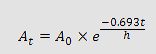Insilicase
deus ex computaGrams to addFinal volumeMaximum concentrationsStock solutionsOD to concOD to primer concProtein in DNA solnCentrifugation calculationsIsotope decayRadioactivity to molarityRadioactivity units conversion

This page is designed to calculate how much radio activity is remaining after a specific number of days. To calculate the current activity enter the compounds original activity, the elapsed time in days since the activity date and the compounds half life press the calculate button.

 Original activity Days elapsed since activity date Compounds half life N/A

The rate of radioactive decay is given by:where:
t is the time in days since the stated activity (A0),
h is the half life of the isotope in days.

### Selected values for commonly used isotopes

 Isotope Half life (days) Energy maximum(MeV) Energy average(MeV) Distance in air 35S 87 beta 0.167 0.049 32cm 32P 14.29 beta 1.710 0.695 730cm 33P 25.3 beta 0.249 0.077 56cm 3H 4492.575 beta 0.0186 0.0057 1cm 14C 2092882.5 beta 0.156 0.049 30cm